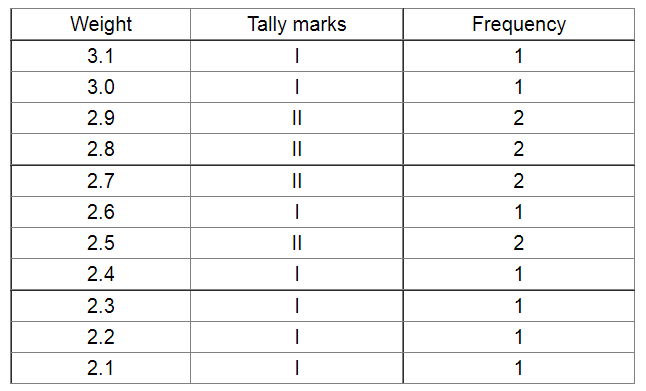# The weights of new born babies (in kg) in a hospital on a particular day are as follows:

Question:

The weights of new born babies (in kg) in a hospital on a particular day are as follows:

2.3, 2.2, 2.1, 2.7, 2.6, 3.0, 2.5, 2.9, 2.8, 3.1, 2.5, 2.8, 2.7, 2.9, 2.4

(i) Rearrange the weights in descending order.

(ii) Determine the highest weight.

(iii) Determine the lowest weight.

(iv) Determine the range.

(v) How many babies were born on that day?

(vi) How many babies weigh below 2.5 kg?

(vii) How many babies weigh more than 2.8 kg?

(viii) How many babies weigh 2.8 kg?

Solution:

The frequency distribution of the weights of new born babies in a hospital on a particular day is represented in the following table:

(i) The weights of the newly born babies in descending order are as follows:(ii) The highest weight is 3.1 kg.

(iii) The lowest weight is 2.1 kg.

(iv) The range is $3.1-2.1$, i.e. $1 \mathrm{~kg}$.

(v) The number of babies born on that day is 15.

(vi) The number of babies whose weights are below 2.5 kg is 4 (i.e. 2.4, 2.3, 2.2, 2.1).

(vii) The number of babies whose weights are more than 2.8 kg is 4 (i.e. 3.1, 3.0, 2.9, 2.9).

(viii) The number of babies whose weight is 2.8 kg is 2.